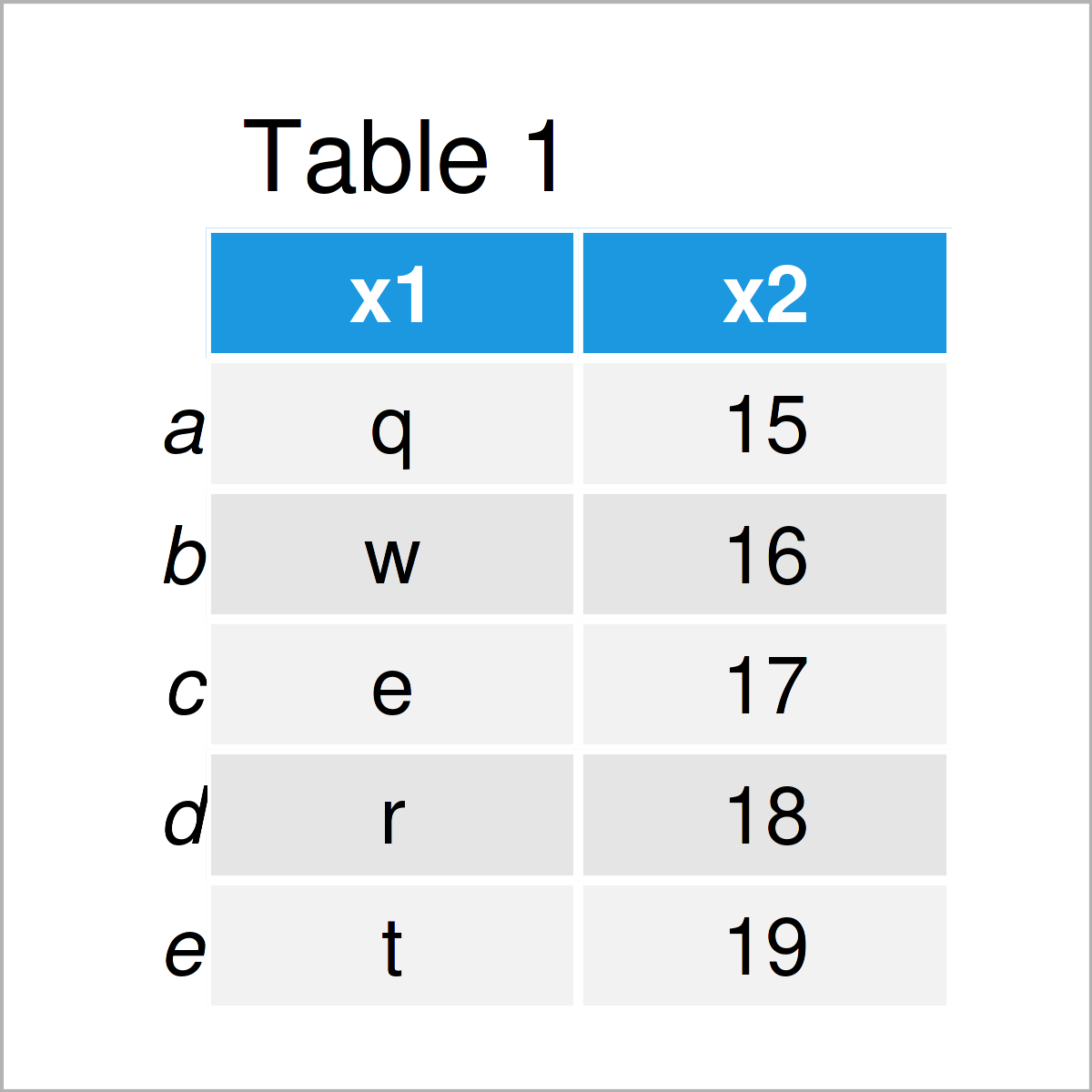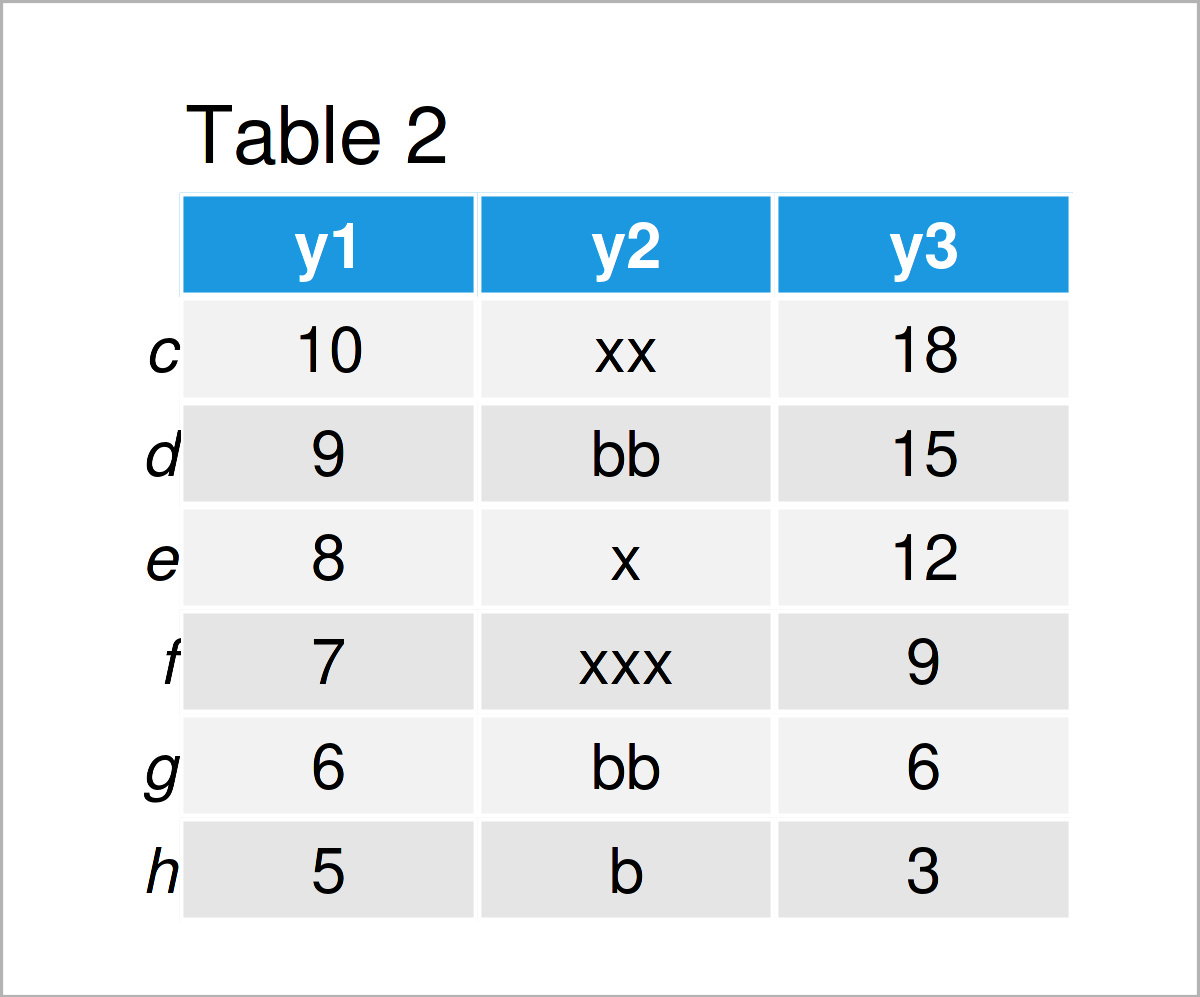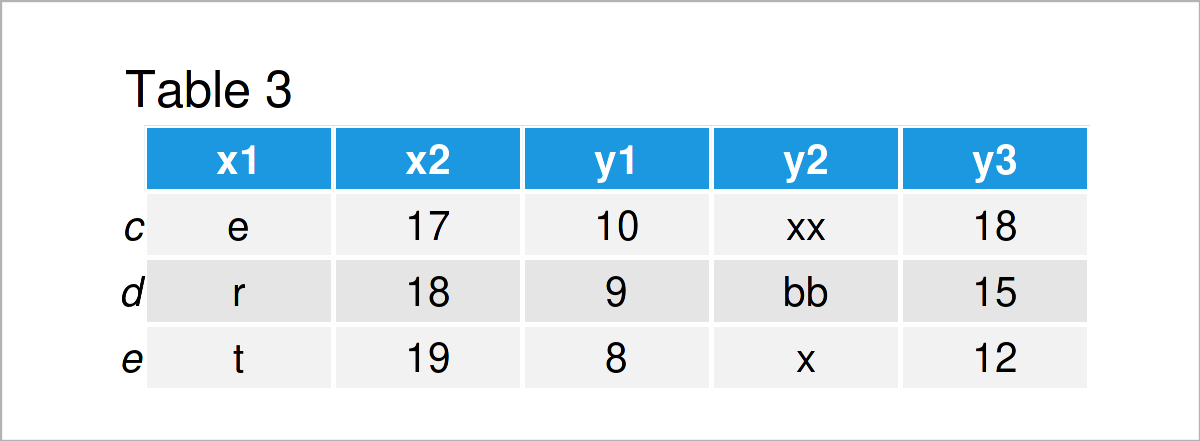# Merge pandas DataFrames based on Index in Python (2 Examples)

In this tutorial, I’ll illustrate how to join two DataFrames based on row indices in the Python programming language.

The post will consist of two examples for the concatenation of two DataFrames based on index values. More precisely, the article consists of the following topics:

Let’s dive right into the examples!

## Example Data & Software Libraries

We first need to load the pandas library:

`import pandas as pd                                    # Load pandas library`

The following DataFrames are used as basement for this Python tutorial:

```data1 = pd.DataFrame({"x1":["q", "w", "e", "r", "t"],  # Create first pandas DataFrame
"x2":range(15, 20)},
index = list("abcde"))
print(data1)                                           # Print first pandas DataFrame``````data2 = pd.DataFrame({"y1":range(10, 4, - 1),          # Create second pandas DataFrame
"y2":["xx", "bb", "x", "xxx", "bb", "b"],
"y3":range(18, 1, - 3)},
index = list("cdefgh"))
print(data2)                                           # Print second pandas DataFrame```Tables 1 and 2 show the structure of our example DataFrames: Both DataFrames contain different columns and values, but partly overlapping row names.

The following examples show how to use these row names to combine our two DataFrames horizontally.

## Example 1: Merge pandas DataFrames based on Index Using Inner Join

Example 1 shows how to use an inner join to append the columns of our two data sets.

For this, we have to apply the merge function, and within the merge function we have to specify the left_index and right_index arguments to be equal to True:

```data_merge1 = pd.merge(data1,                          # Inner join based on index
data2,
left_index = True,
right_index = True)
print(data_merge1)                                     # Print merged DataFrame```The output of the previous Python code is shown in Table 3 – A horizontally stacked pandas DataFrame containing the shared row indices of our two input DataFrames.

As you can see, we have removed several rows from our example DataFrames, since the indices of these DataFrames are not fully overlapping.

Let’s perform another join to keep as much data as possible.

## Example 2: Merge pandas DataFrames based on Index Using Outer Join

Example 2 illustrates how to use an outer join to retain all rows of our two input DataFrames.

For this, we have to specify the how argument within the merge function to be equal to “outer”. Besides this, we can use the same syntax as in Example 1 to add our two DataFrames together:

```data_merge2 = pd.merge(data1,                          # Outer join based on index
data2,
left_index = True,
right_index = True,
how = "outer")
print(data_merge2)                                     # Print merged DataFrame```In Table 4 you can see that we have created a new union of our two pandas DataFrames. This time, we have kept all rows and inserted NaN values in case a row index was only available in one of the input DataFrames.

By the way, please note that in this tutorial we have merged only two DataFrames. However, we could use this approach to merge multiple DataFrames in Python as well.

## Video & Further Resources

Do you want to learn more about the concatenation of two DataFrames based on index values? Then I recommend having a look at the following video on my YouTube channel. In the video, I’m explaining the Python programming code of the present article:

Please accept YouTube cookies to play this video. By accepting you will be accessing content from YouTube, a service provided by an external third party.If you accept this notice, your choice will be saved and the page will refresh.

Furthermore, you may want to read the related posts on https://statisticsglobe.com/:

In this Python tutorial you have learned how to merge two DataFrames based on index values. Please let me know in the comments below, in case you have additional questions.

Subscribe to the Statistics Globe Newsletter